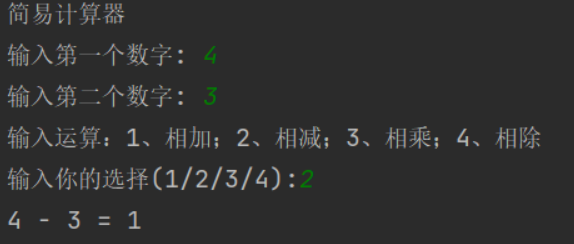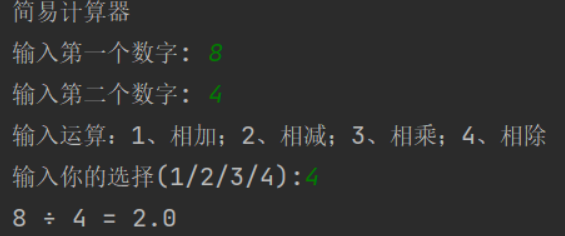# Python 之简易计算器的制作

• 2022 年 9 月 30 日
四川
• 本文字数：1146 字

阅读完需：约 4 分钟

# 1.解题的思路

• 请用户输入待运算的两个数字

• 请用户选择运算方法

• 将运行结果展示出来

2.方法

### 方法一

print("简易计算器")def add(x, y):    return x + ydef subtract(x, y):    return x - ydef multiply(x, y):    return x * ydef divide(x, y):    return x / ynum1 = int(input("输入第一个数字: "))num2 = int(input("输入第二个数字: "))print("输入运算：1、相加；2、相减；3、相乘；4、相除")choice = input("输入你的选择(1/2/3/4):")if choice == '1':    print(num1, "+", num2, "=", add(num1, num2))elif choice == '2':    print(num1, "-", num2, "=", subtract(num1, num2))elif choice == '3':    print(num1, "×", num2, "=", multiply(num1, num2))elif choice == '4':    print(num1, "÷", num2, "=", divide(num1, num2))else:    print("非法输入")### 方法二

print("简易计算器")num1 = int(input("输入第一个数字: "))num2 = int(input("输入第二个数字: "))print("输入运算：1、相加；2、相减；3、相乘；4、相除")choice = input("输入你的选择(1/2/3/4):")if choice == '1':   print(num1, "+", num2, "=", num1 + num2)elif choice == '2':   print(num1, "-", num2, "=", num1 - num2)elif choice == '3':   print(num1, "×", num2, "=", num1 * num2)elif choice == '4':   print(num1, "÷", num2, "=", num1 / num2)else:   print("非法输入")### 向阳逐梦

InfoQ签约作者、阿里云“乘风者计划”签约博主

## 评论